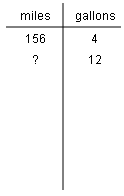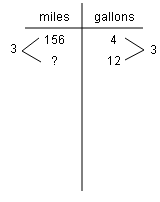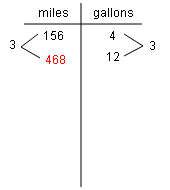### Home > CC2 > Chapter 5 > Lesson 5.3.4 > Problem5-139

5-139.

If you can travel $156$ miles on $4$ gallons of gasoline, how far can you travel on $12$ gallons? How many miles on $6$ gallons? A diagram may help you with your reasoning. Show your work and explain your thinking.

Since each gallon of gasoline gives the same amount of mileage, use a common ratio for the miles per gallon. 2 column chart: labeled as follows: Row 1, miles and gallons, Row 2: 156 and 4, Row 3: question mark and 12.

The ratio between $4$ and $12$ gallons is $3$ so this ratio also translates to the mileage. Added to the chart, between the second and third rows, in both columns, a change of 3.

$468$ miles. Use this same strategy to figure out the mileage for $6$ gallons. Added to the chart, Row 3, first column, 468.